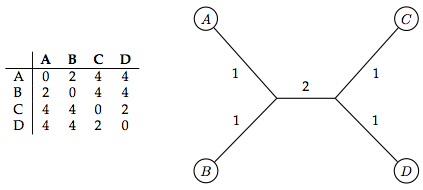# Glossary

## Distance-based phylogeny

Distance-based phylogeny aims to form an evolutionary tree on a collection of taxa by using a function that quantifies a distance measure between pairs of taxa. For example, if our taxa are represented by genetic strings, then Hamming or edit distance may be applied.

Once a particular metric has been selected, the distances between pairs of taxa are consolidated into a distance matrix $D$, in which $D_{j,k}$ represents the distance between the $j$th and $k$th taxon. The algorithmic challenge is to reconstruct a binary tree whose leaves correspond to taxa and for which the (weighted) distance between two leaves in the tree corresponds to the evolutionary distance between the two taxa.

For example, the figure below shows a consistent distance matrix on four taxa along with an unrooted binary tree modeling this distance matrix.